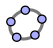# Applets DirectoryHenri Picciotto

Applets are small, single-purpose computer programs, usually available on the Web through a browser. For this site, I built the applets in GeoGebra, with the goal of making various mathematical ideas more visual and more interactive. However you cannot expect them to teach the concepts they illustrate! They are most effective if they are part of a multidimensional pedagogy that gets students to engage intellectually rather than watch passively.

You can use the applets online, and/or download the corresponding GeoGebra files and modify them to better fit your needs.

Some links below are to a single applet, others are to a launch page with links to several applets.

### Algebra

Changing a, b, c in y=ax^2+bx+c

Completing the Square

Complex Number Arithmetic Games

Constant Sums, Constant Products

Difference of Squares

Multiplying binomials

Off and On, understanding linear inequalities

Squaring a Binomial

Stairs, slope puzzles

Trinomial: Multiple Representations

Virtual Geoboard

Slices

Rectangles

### Functions

Doctor Dimension, exploring rate of change

Function Diagrams, a parallel axes representation

Sine Curve

Iterating Linear Functions:
with a table
with a 'cobweb' graph

Iterating f(x)=rx(1-x) (in two representations)

Bifurcation graph for f(x)=rx(1-x)

### Geometry

Area of a Circle

Dilation

Geometry of the Parabola

Isometries (Rigid Motions)

Only four kinds

Making Triangles

SSA

Pythagorean Theorem

Sum of the Angles in a Triangle

Symmetry with Pattern Blocks

Tri, Tri Again (inscribed circle iteration)

Virtual Circle Geoboard

Virtual Geoboard

Virtual Pentominoes

Virtual Tangrams

### Other

Parallelogram area

Pent, a two-person strategy game

Penumbra, some of the geometry of eclipses

Spirograph, exploring symmetric figures created by a circle in a circle

Virtual Grid Paper

As I create more applets, I'll try to remember to add links in this directory.